## Drill pipe pulled to lose hydrostatic pressureYou previously learn about hydrostatic pressure lose due to pulling out of hole . This article will use the same concept but we will determine how many feet of drill pipe pulled to lose certain amount of hydrostatic pressure in well bore.

## Oilfield Unit

Pipe pulled, ft = (hydrostatic pressure loss in psi × (casing cap in bbl/ft – pipe displacement in bbl/ft)) ÷ (mud weight in ppg × 0.052 × pipe displacement in bbl/ft)

Example: Determine the FEET of drill pipe that must be pulled to lose 200 psi overbalance using the following data:

Hydrostatic pressure loss = 200 psi
Casing capacity = 0.0873 bbl/ft
Pipe displacement = 0.01876 bbl/ft
Mud weight = 12.0 ppg
Pipe pulled  = 200 psi × (0.0873 – 0.01876) ÷ (12.0 ppg × 0.052 × 0.01876)
Pipe pulled = 1171 ft
You need to pull 1171 ft of dry pipe to lose 200 psi hydrostatic pressure.

## Metric Unit

Pipe pulled, m = (hydrostatic pressure loss in KPa× (casing cap in m3/m- pipe displacement in m3/m-)) ÷ (mud weight in kg/m3 × 0.00981× pipe displacement in m3/m)

Example: Determine how many meters of drill pipe that must be pulled to lose 1400 KPa overbalance using the following data:

Hydrostatic pressure loss = 1400 KPa
Casing capacity = 0.04554 m3/m
Pipe displacement = 0.00979 m3/m
Mud weight = 1440 kg/m3
Pipe pulled  = 1400× (0.04554 – 0.00979 ) ÷ (1440 × 0.00981 × 0.00979 )
Pipe pulled = 362 m
You need to pull 362 m of dry pipe to lose 1400 KPa hydrostatic pressure.

Please find the Excel Spreadsheet to calculate how many feet  or meter of drill pipe pulled to lose certain amount of hydrostatic pressure in well bore.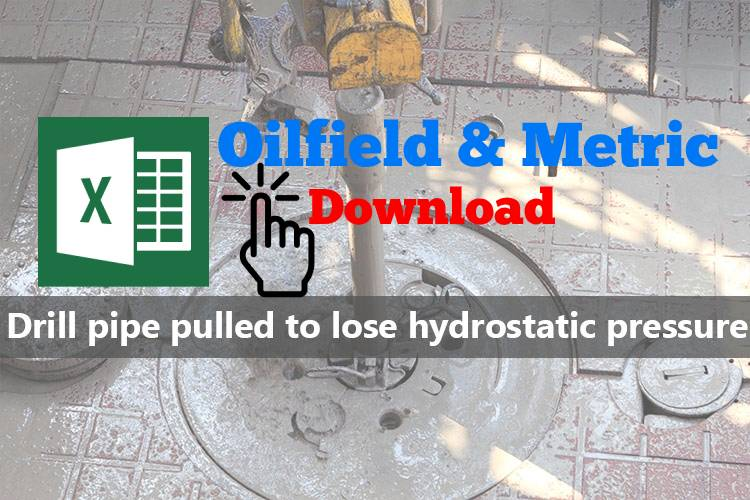Ref books:

Lapeyrouse, N.J., 2002. Formulas and calculations for drilling, production and workover, Boston: Gulf Professional publishing.

Bourgoyne, A.J.T., Chenevert , M.E. & Millheim, K.K., 1986. SPE Textbook Series, Volume 2: Applied Drilling Engineering, Society of Petroleum Engineers.

Mitchell, R.F., Miska, S. & Aadny, B.S., 2011. Fundamentals of drilling engineering, Richardson, TX: Society of Petroleum Engineers.

## Hydrostatic Pressure (HP) Decreases When POOHWhen pulling out of hole, volume of steel will be out of hole and mud volume will replace the steel volume.  If we don’t fill hole, hydrostatic pressure will decrease.  This topic shows you how to calculate hydrostatic pressure loss while pulling out of hole without filling the wellbore.  Moreover, there is the Excel sheet for calculating pressure decrease due to pulling out of hole.

## Oilfield Unit

Step 1: Determine Total Volume of Steel Out of Hole

Total Volume of Steel Out of Hole = Length  of pipe pulled out × Pipe Displacement

Where,

Total Volume of Steel Out of Hole in bbl

Length  of pipe pulled out in ft

Pipe Displacement in bbl/ft

Step 2: Determine Hydrostatic Pressure Decrease

Hydrostatic Pressure Decrease = (Total Volume of Steel Out of Hole × 0.052 × mud weight) ÷ (casing capacity – pipe displacement)

Where,

Hydrostatic Pressure Decrease in psi

Total Volume of Steel Out of Hole in bbl

mud weight in ppg

casing capacity in bbl/ft

pipe displacement in bbl/ft

Example: Determine the hydrostatic pressure decrease when pulling pipe out of the hole:

Number of stands pulled = 10
Pipe displacement = 0.0055 bbl/ft
Average length per stand = 91 ft
Casing capacity = 0.0873 bbl/ft
Mud weight = 12.0 ppg

Step 1: Determine of pipe displacement in Barrels = 10 stands × 91 ft/std × 0.0055 bbl/ft displaced

Total Volume of Steel Out of Hole = 5.01 bbl

Step 2: Determine HP, psi decrease = 5.01 barrels × 0.052 × 12.0 ppg ÷ (0.0873 bbl/ft – 0.0055 bbl/ft)

Hydrostatic pressure decrease = 38.2 psi

## Metric Unit

Step 1: Determine Total Volume of Steel Out of Hole

Total Volume of Steel Out of Hole = Length  of pipe pulled out × Pipe Displacement

Where,

Total Volume of Steel Out of Hole in m3

Length  of pipe pulled out in m

Pipe Displacement in m3 /m

Step 2: Determine Hydrostatic Pressure Decrease

Hydrostatic Pressure Decrease = (Total Volume of Steel Out of Hole × 0.00981 × mud weight) ÷ (casing capacity – pipe displacement)

Where,

Hydrostatic Pressure Decrease in KPa

Total Volume of Steel Out of Hole in m3

mud weight in kg/m3

casing capacity in m3 /m

pipe displacement in m3 /m

Example: Determine the hydrostatic pressure decrease when pulling pipe out of the hole:

Number of stands pulled = 10
Pipe displacement = 0.00287 m3 /m
Average length per stand = 30 m
Casing capacity = 0.04554 m3 /m
Mud weight = 1440 kg/m3

Step 1: Determine of pipe displacement in m3 = 10 stands × 30 m/std × 0.00287 m3 /m pipe displacement

Total Volume of Steel Out of Hole 0.86  m3

Step 2: Determine HP, psi decrease = 0.86  m3 × 0.00981 × 1440 kg/m3 ÷ (0.04554 m3 /m- 0.00287 m3 /m)

Hydrostatic pressure decrease = 285 KPa

Please find the Excel sheet for calculating pressure decrease due to pulling out of hole.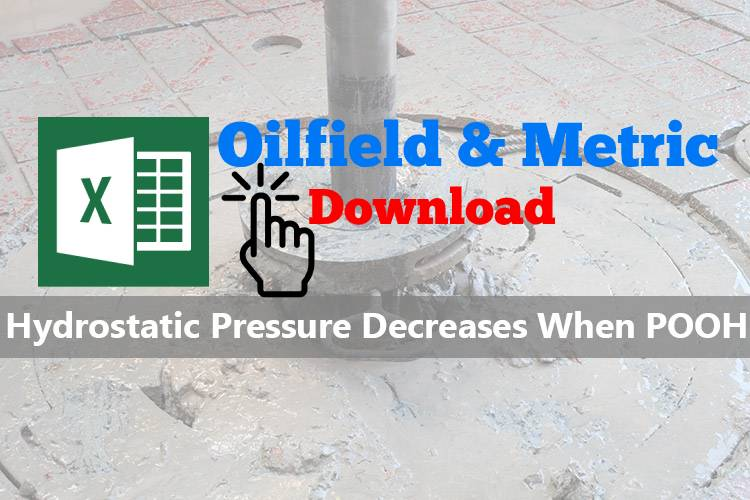Ref books:

Lapeyrouse, N.J., 2002. Formulas and calculations for drilling, production and workover, Boston: Gulf Professional publishing.

Bourgoyne, A.J.T., Chenevert , M.E. & Millheim, K.K., 1986. SPE Textbook Series, Volume 2: Applied Drilling Engineering, Society of Petroleum Engineers.

Mitchell, R.F., Miska, S. & Aadny, B.S., 2011. Fundamentals of drilling engineering, Richardson, TX: Society of Petroleum Engineers.

## Pipe Displacement Calculation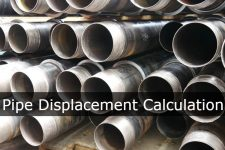Pipe displacement, normally in bbl/ft or m3/m, is steel volume  per length of steel pipe.  When we either pull out of hole or trip in hole for any kind of pipes such as drill pipe, casing or tubing, you should know how much fluid to displace steel volume.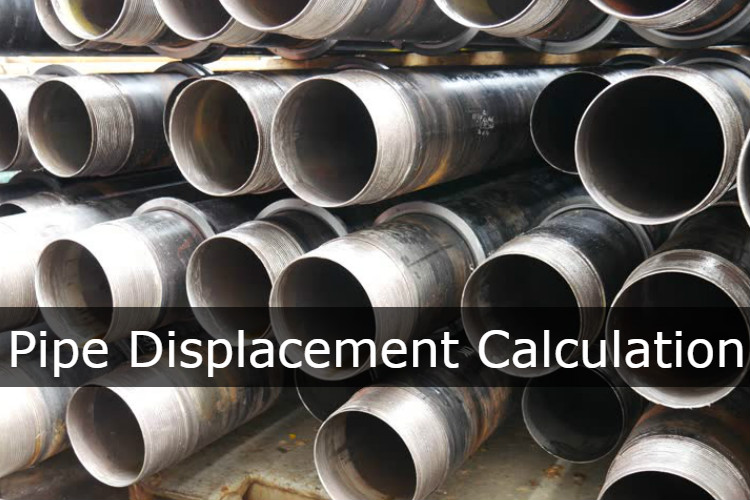For example, when we pull out of hole, a trip sheet must be monitored all time. We must know how much fluid will fill the hole each stand of drill pipe pulled out. If the volume of displacement less than theoretical displacement value, we may have problem due to swabbing formation into wellbore.

This article will demonstrate how to calculate plain pipe displacement with this following formula:

## Oilfield Unit

Pipe Displacement in bbl/ft = (OD2 – ID2 ) ÷ 1029.4

Where;
OD in inch
ID in inch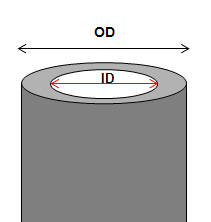Where,
OD is out side diameter of pipe in inch.
ID is inside diameter of pipe in inch.

This formula is for plain pipe diplacment such as casing and tubing. It is not for closed-end displacement. It’s not accurate enough for drill pipe because this formula does not account for tool joint displacement therefore you need drill pipe specification sheet for its displacement.

Example: Determine pipe displacement in bbl/ft of 9-5/8” casing 40 ppf, OD = 9.625 in, ID = 8.835 in

Pipe Displacement of 9-5/8” casing 40 ppf in bbl/ft = (9.6252 – 8.8352 ) ÷1029.4
Pipe Displacement of 9-5/8” casing 40 ppf in bbl/ft = 0.01417 bbl/ft

## Metric Unit

Annular capacity in m3/m =  (OD2 – ID2) ÷1,273,240

Where;
OD in mm
ID in mm

Example: Determine pipe displacement in bbl/ft of 9-5/8” casing 40 ppf, OD = 244.475 mm, ID = 244.409 mm

Pipe Displacement of 9-5/8” casing 40 ppf in m3/m = (244.4752 – 244.4092 ) ÷1,273,240
Pipe Displacement of 9-5/8” casing 40 ppf in m3//m = 0.0074

Ref books:

Lapeyrouse, N.J., 2002. Formulas and calculations for drilling, production and workover, Boston: Gulf Professional publishing.

Bourgoyne, A.J.T., Chenevert , M.E. & Millheim, K.K., 1986. SPE Textbook Series, Volume 2: Applied Drilling Engineering, Society of Petroleum Engineers.

Mitchell, R.F., Miska, S. & Aadny, B.S., 2011. Fundamentals of drilling engineering, Richardson, TX: Society of Petroleum Engineers.